• lstm神经网络模型
千次阅读
2020-04-21 23:53:08

## Step1：导入包

import numpy as np
import  pandas as pd
import matplotlib.pyplot as plt
from sklearn.preprocessing import MinMaxScaler
import os
import tensorflow as tf
from tensorflow import keras


## Step2：设置GPU的使用率可按需增长

# set GPU
tf.debugging.set_log_device_placement(True)
gpus = tf.config.experimental.list_physical_devices('GPU')
tf.config.experimental.set_visible_devices(gpus, 'GPU')
for gpu in gpus:
tf.config.experimental.set_memory_growth(gpu, True)
print(len(gpus))
logical_gpus = tf.config.experimental.list_logical_devices('GPU')
print(len(logical_gpus))


## Step3：读取数据

# read data-sensor.csv
pd_value = dataframe.values


## Step4：设置参数

look_back = 4
features = 28


look_back：一匹数据所含的数据个数
features：每个数据所拥有的特征数

## Step5：构建数据集

### 切分数据

# ========= split dataset ===================
train_size = int(len(pd_value) * 0.8)
trainlist = pd_value[:train_size]
testlist = pd_value[train_size:]


### 创建数据集

# ========= numpy train ===========
def create_dataset(dataset, look_back):
#这里的look_back与timestep相同
dataX, dataY = [], []
for i in range(len(dataset)-look_back-1):
a = dataset[i:(i+look_back)]
dataX.append(a)
dataY.append(dataset[i + look_back])
return numpy.array(dataX),numpy.array(dataY)
#训练数据太少 look_back并不能过大
trainX,trainY  = create_dataset(trainlist,look_back)
testX,testY = create_dataset(testlist,look_back)

# ========== set dataset ======================
trainX = numpy.reshape(trainX, (trainX.shape, trainX.shape, features))
testX = numpy.reshape(testX, (testX.shape, testX.shape , features))


## Step6：模型构建及训练

### 构建LSMT神经网络模型

# create and fit the LSTM network
model = tf.keras.Sequential()
model.add(tf.keras.layers.LSTM(64, activation='relu', return_sequences=True, input_shape=(look_back, features)))

model.summary()


### 训练模型并保存

history = model.fit(trainX, trainY, validation_data=(testX, testY),epochs=15, verbose=1).history
model.save("lstm-model.h5")


### 画图查看模型学习情况

plt.plot(history['loss'], linewidth=2, label='Train')
plt.plot(history['val_loss'], linewidth=2, label='Test')
plt.legend(loc='upper right')
plt.title('Model loss')
plt.ylabel('Loss')
plt.xlabel('Epoch')
#plt.ylim(ymin=0.70,ymax=1)
plt.show()


## Step7：模型检验

### 训练检验

trainPredict = model.predict(trainX)
testPredict = model.predict(testX)


### 画图检验

训练集情况

plt.plot(trainY[:100,1])
plt.plot(trainPredict[:100,1])
plt.show()


测试集情况

plt.plot(testY[:100,1])
plt.plot(testPredict[:100,1])
plt.plot()


## Step8：进行预测

### 设置训练集

# set predict_data
predict_begin = 1
predict_num = 100
predict_result = np.zeros((predict_num+look_back,features),dtype=float)
for i in range(look_back):
predict_result[i] = testX[-predict_begin:][0,i]


预测后100个数据情况，从最后一个数据开始进行时间序列预测。构建预测结果变量predict_result

### 进行预测

# predict
for i in range(predict_num):
begin_data = np.reshape(predict_result[i:i+look_back,], (predict_begin, look_back, features))
predict_data = model.predict(begin_data)
predict_result[look_back+i] = predict_data
buff = predict_result[i+1:i+look_back]
predict_call_back = np.append(buff,predict_data,axis=0)


构建滚动预测数据，每次取四行数据进行预测。
预测后，将原预测前的三行数据与预测后的结果进行拼接，组成四行数据进行下一轮的预测。

### 画图展示

# show plot
plt.plot(predict_result[-predict_num:,5])
plt.plot()


## 完整代码

import numpy as np
import  pandas as pd
import matplotlib.pyplot as plt
from sklearn.preprocessing import MinMaxScaler
import os
import tensorflow as tf
from tensorflow import keras

# set GPU
tf.debugging.set_log_device_placement(True)
gpus = tf.config.experimental.list_physical_devices('GPU')
tf.config.experimental.set_visible_devices(gpus, 'GPU')
for gpu in gpus:
tf.config.experimental.set_memory_growth(gpu, True)
print(len(gpus))
logical_gpus = tf.config.experimental.list_logical_devices('GPU')
print(len(logical_gpus))

pd_value = dataframe.values

# ========= split dataset ===================
train_size = int(len(pd_value) * 0.8)
trainlist = pd_value[:train_size]
testlist = pd_value[train_size:]

look_back = 4
features = 28
step_out = 1

# ========= numpy train ===========
def create_dataset(dataset, look_back):
#这里的look_back与timestep相同
dataX, dataY = [], []
for i in range(len(dataset)-look_back-1):
a = dataset[i:(i+look_back)]
dataX.append(a)
dataY.append(dataset[i + look_back])
return numpy.array(dataX),numpy.array(dataY)
#训练数据太少 look_back并不能过大
trainX,trainY  = create_dataset(trainlist,look_back)
testX,testY = create_dataset(testlist,look_back)

# ========== set dataset ======================
trainX = numpy.reshape(trainX, (trainX.shape, trainX.shape, features))
testX = numpy.reshape(testX, (testX.shape, testX.shape , features))

# create and fit the LSTM network
model = tf.keras.Sequential()
model.add(tf.keras.layers.LSTM(64, activation='relu', return_sequences=True, input_shape=(look_back, features)))

model.summary()

history = model.fit(trainX, trainY, validation_data=(testX, testY),epochs=15, verbose=1).history
model.save("lstm-model.h5")

plt.plot(history['loss'], linewidth=2, label='Train')
plt.plot(history['val_loss'], linewidth=2, label='Test')
plt.legend(loc='upper right')
plt.title('Model loss')
plt.ylabel('Loss')
plt.xlabel('Epoch')
#plt.ylim(ymin=0.70,ymax=1)
plt.show()

trainPredict = model.predict(trainX)
testPredict = model.predict(testX)

plt.plot(trainY[:100,1])
plt.plot(trainPredict[:100,1])
plt.show()

plt.plot(testY[:100,1])
plt.plot(testPredict[:100,1])
plt.plot()

# set predict_data
predict_begin = 1
predict_num = 100
predict_result = np.zeros((predict_num+look_back,features),dtype=float)
for i in range(look_back):
predict_result[i] = testX[-predict_begin:][0,i]

# predict
for i in range(predict_num):
begin_data = np.reshape(predict_result[i:i+look_back,], (predict_begin, look_back, features))
predict_data = model.predict(begin_data)
predict_result[look_back+i] = predict_data
buff = predict_result[i+1:i+look_back]
predict_call_back = np.append(buff,predict_data,axis=0)

# show plot
plt.plot(predict_result[-predict_num:,5])
plt.plot()


参考文档：

简单粗暴LSTM：LSTM进行时间序列预测

Kesci： Keras 实现 LSTM——时间序列预测

【tensorflow2.0】处理时间序列数据

时间序列预测09：如何开发LSTM实现时间序列预测详解 03 Multi-step LSTM

Python：利用LSTM预测时间序列数据

python利用LSTM进行时间序列分析预测

LSTM时间序列预测及网络层搭建

LSTM与Prophet时间序列预测实验BraveY神经网络 python tensorflow lstm
更多相关内容
• 经过与传统回归模型和传统神经网络模型进行实验对比，实验显示LSTM拟合效果最佳，另外LSTM模型对同一趋势上的预测效果有明显优势。通过使用LSTM模型捕获数据中存在的时序依赖关系，能够更准确地对交通事故安全水平...
• 基于LSTM神经网络模型的疫情发展趋势预测。别采用经典传染病动力学模型SEIR和LSTM神经网络实现，通过控制模型参数来改变干预程度，体现防控的意义。同时利用LSTM递归神经网络时间序列预测算法对疫情发展趋势进行预测...
• 基于多变量LSTM神经网络模型的风暴潮临近预报.pdf
• 基于LSTM神经网络模型预测北京PM2.5排放量预测 代码实现 import tensorflow as tf import numpy as np import pandas as pd import matplotlib.pyplot as plt from tensorflow.keras import layers from sklearn....
• 使用PYthon的keras包来构建LSTM模型（请自行安装keras包)，用疫情前七天的数据作为训练集构建LSTM神经网络实现对疫情的预测。
• 使用LSTM神经网络进行时间序列数据预测分析。 基于Tensorflow框架、Kerase接口开发网络模型。 包含数据清洗，数据特征提取，数据建模，数据预测。 包含洗发水销量预测、空气质量预测等实际案例。 LSTM单变量、Multi-...
• LSTM神经网络模型在GPS变形监测中的应用研究.pdf
• 计算机研究 -基于层次聚类的LSTM神经网络模型在江苏省降水量预测中的应用.pdf
• 基于LSTM神经网络的美股股指价格趋势预测模型的研究 基于LSTM神经网络的美股股指价格趋势预测模型的研究 基于LSTM神经网络的美股股指价格趋势预测模型的研究 基于LSTM神经网络的美股股指价格趋势预测模型的研究 基于...
• 基于疫情时空分布特征构建GA-BP神经网络预测模型，同时利用LSTM递归神经网络时间序列预测算法对疫情发展趋势进行预测。本研究的数据收集时间为2020年1月23日-2020年4月30日，疫情时空分布特征（温度，湿度，风向）的...
• 在pytorch下，以数万首唐诗为素材，训练双层LSTM神经网络，使其能够以唐诗的方式写诗。 代码结构分为四部分，分别为 1.model.py，定义了双层LSTM模型 2.data.py，定义了从网上得到的唐诗数据的处理方法 3.utlis.py ...
• 基于CNN-LSTM混合神经网络模型的短期负荷预测方法 基于CNN-LSTM混合神经网络模型的短期负荷预测方法 基于CNN-LSTM混合神经网络模型的短期负荷预测方法 基于CNN-LSTM混合神经网络模型的短期负荷预测方法 基于CNN-LSTM...
• 在谈人工神经网络模型之前我们先来了解一下生理上的神经网络。 下面是个对比图： Neural Science Computer Science 人工神经网络实际上就是模仿人类的神经元之间连结，就像图灵模型也是基于人类运算行为而提出的一...

# LSTM的学习

### 学习目标：

				  1·理解什么是人工神经网络。
2·深入理解LSTM（长短期记忆网络）
3·Code


### 浅析人工神经网络：

在谈人工神经网络模型之前我们先来了解一下生理上的神经网络。
下面是一张对比图：
Neural ScienceComputer Science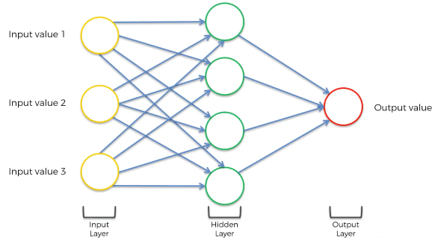人工神经网络实际上是模仿人类的神经元网络的搭建，就像最初图灵模型也是基于人类运算行为而提出的一个模型。在人体中，当感受器受到刺激的时候会产生神经冲动，产生信号。神经元之间通过突起相互连结，实现信息的传递。但是医学上发现，信息传入细胞核之后并不是直接传给下一个神经元，而是需要达到某一特定”值“，被激活之后才能传给其他神经元。所以我们在模仿的时候会引入激活函数（高度非线性的）。另外，对于相同的信息，经过不同的”路线“传递，会出现不同程度的信息损失。因此，我们还会引入权重这一概念。所以人工神经元的工作流程就如下所示：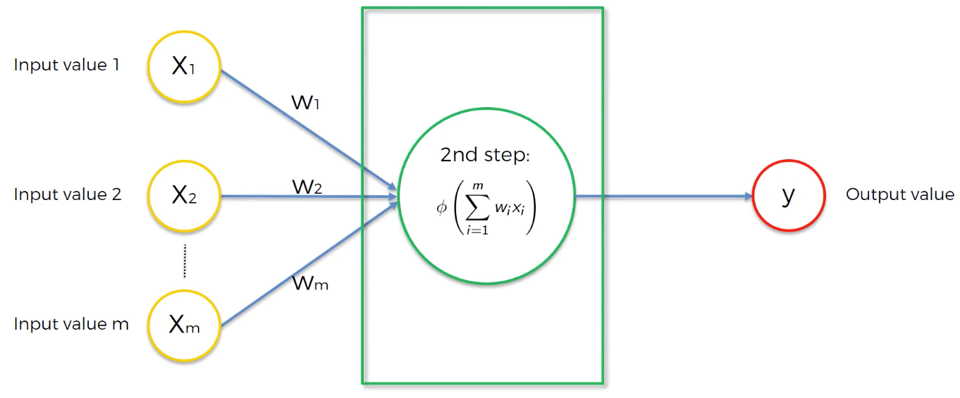外界的输入乘上相应的权重就是该细胞核所获取的信息，信息累加之后由激活函数判断是否输出。
激活函数是信息传递比较重要的一环，但是我们不知道这个高度非线性的函数到底是什么，我们只能去猜这个函数，我们流行的“猜法”有如下四种形式：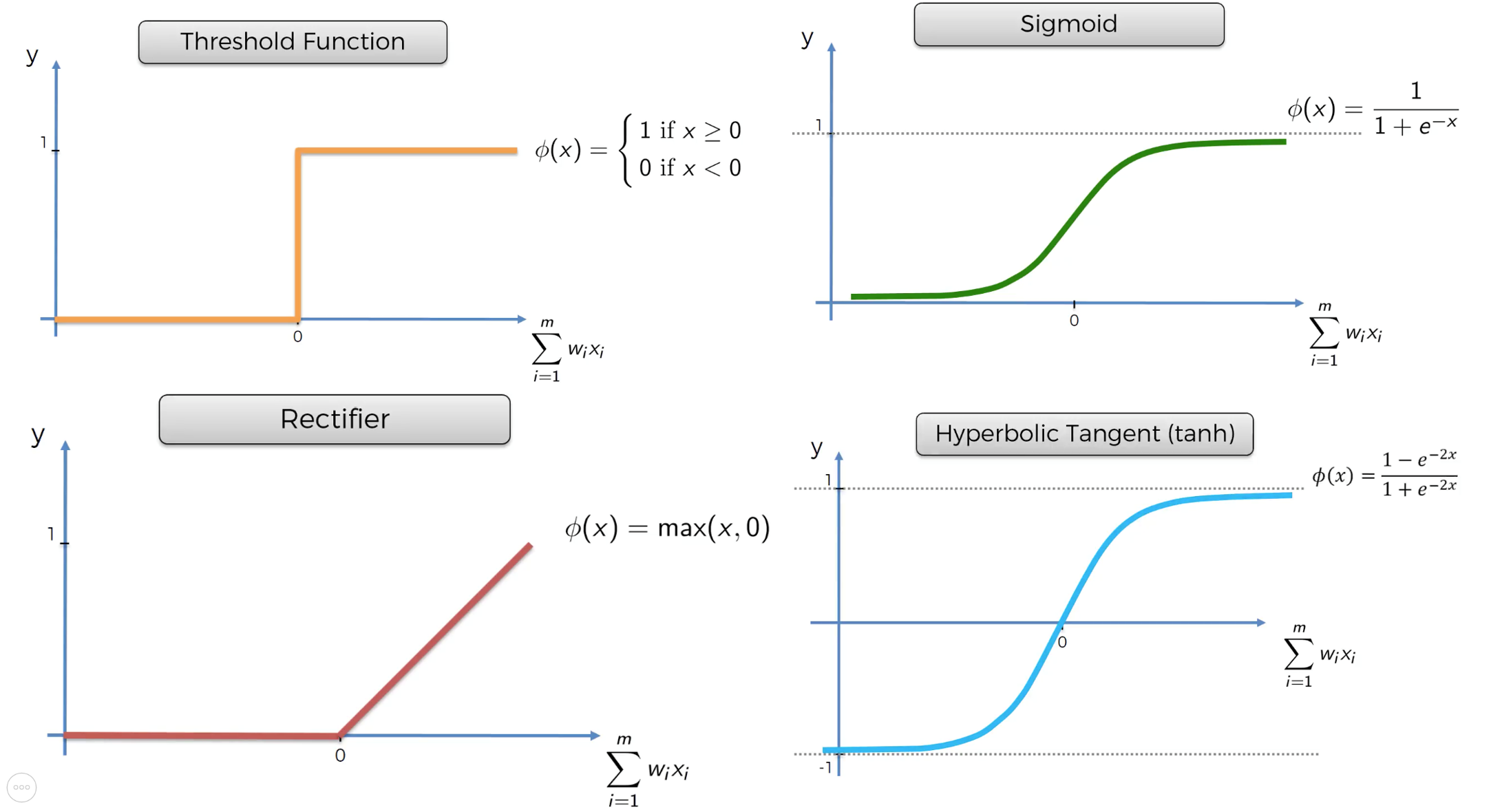当我们用sigmoid去构建网络的时候就可以发现，其实LogisticRegression是最简单的人工神经网络。在使用过程中，我们搭建复杂的人工神经网络，是基于每一个简单的人工神经元，通过排列、组合、连接完成的，本源其实是一样的，本质的东西是不会改变的。
我们在后面的代码coding部分会详细讲解如何使用。
下面是简单的全连接神经网络模型，每一列我们称之为一个神经网络层。由此我们可以具象地看到信息传递的路线的差别和信息的丢失。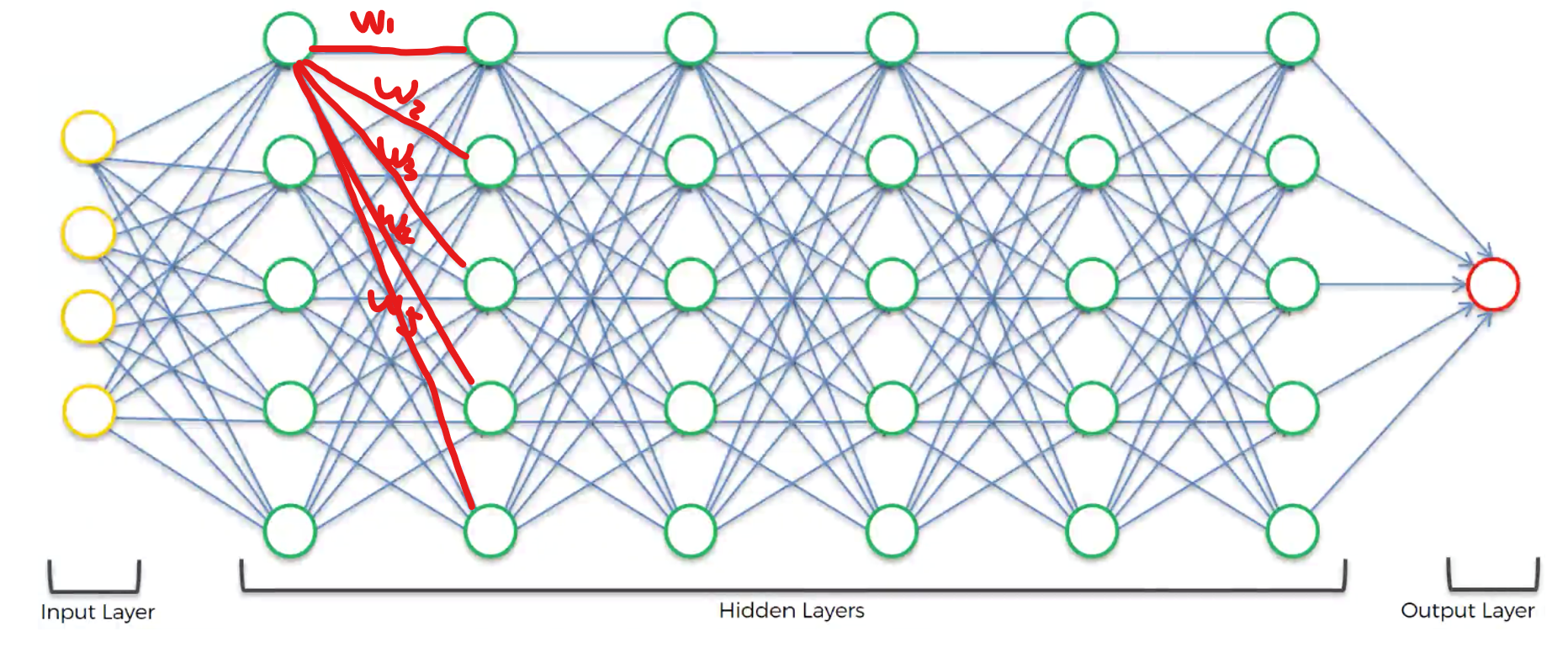### 理解了什么是人工神经网络，我们接下来进行学习一个基础模型解决有关 时间序列问题——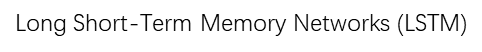先上概览图：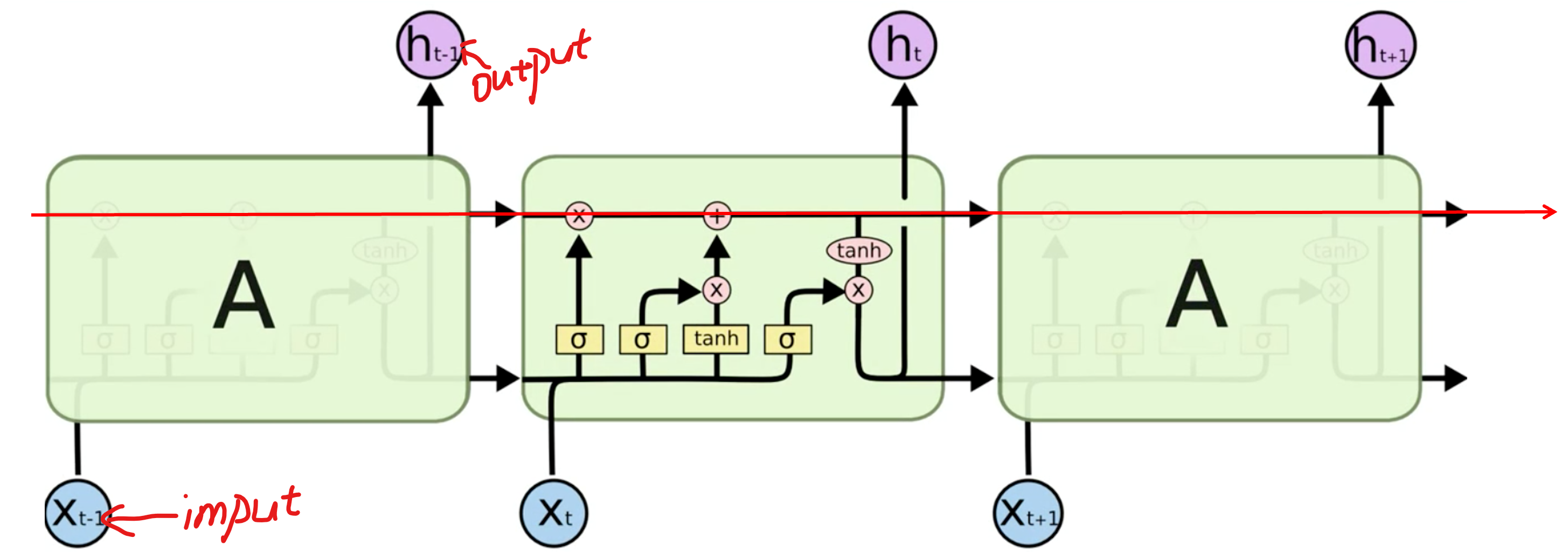LSTM模型在时间轴上是连续的，每一个A都表示某个时间点，信息是有继承的。该模型的处理数据的方式也是模拟人在处理时间序列的方式。
我们可以看到这其中，总共有两条时间序列：
下面这一条时间序列代表的输入=观测数据的输入+前一时刻的输出。
上面这一时间序列是知识、信息的继承，我们来看一看他是怎么运行的：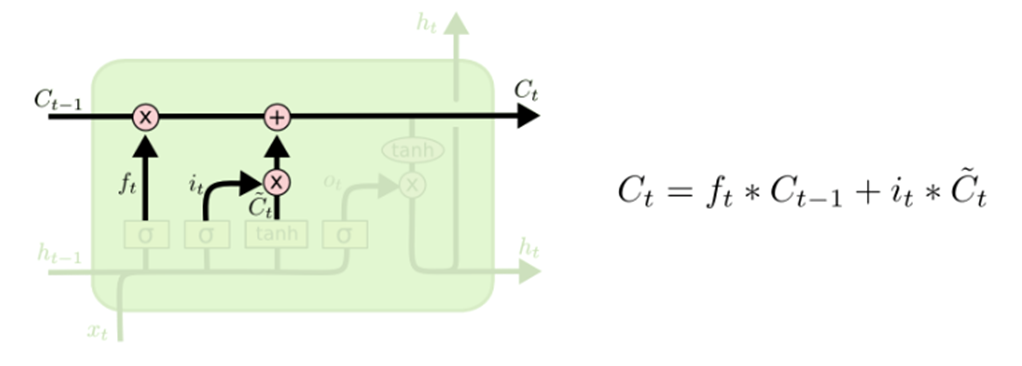Ct-1是该模型在t-1时刻的知识状态，C~t是在输入新的观测之后新获得的信息，分别乘上相应的权重参数之后相加即可得到t时刻的知识状态。这个权重参数就决定了你在这些时刻所保留的知识。打个比方，如果是一个经验主义者，那么他的Ft就会偏大，不太相信新的观测，如果是一个现实主义者那么他的It就会偏大。实际上我们只要把这个等式理解明白了，我们就差不多弄清楚LSTM的原理了。

### 我们接下来讲讲上述等式的各个变量：）

首先是Ft的计算，因为此值是一个百分比数值，所以我们偏向于使用sigmoid激活函数。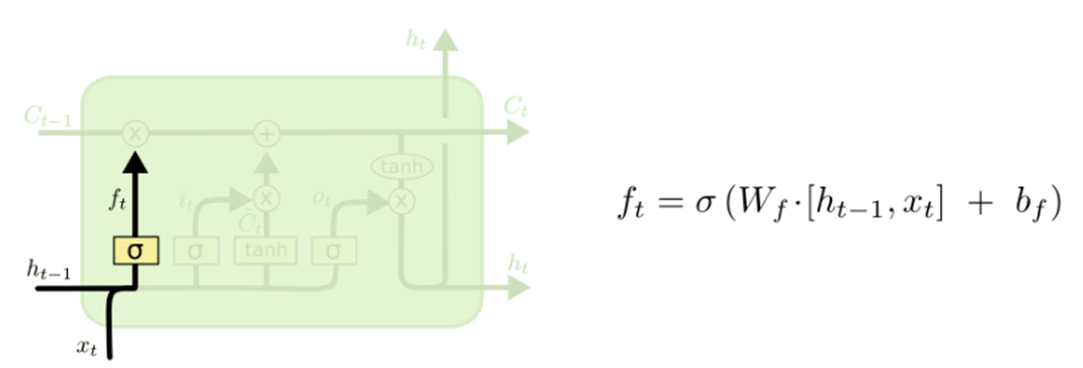Ft的输入= (对应权重) * [(t-1时刻的输出) concat (t时刻的新输入)] 最后套上激活函数。我们还可以看到该等式后面还有一项bf，其实这一项对于帮助我们理解LSTM并没有太大的帮助，只是担心模型太过复杂或者数据过于简单导致的模型过拟合，所加上的随机扰动（白噪音）。
其次是CtIt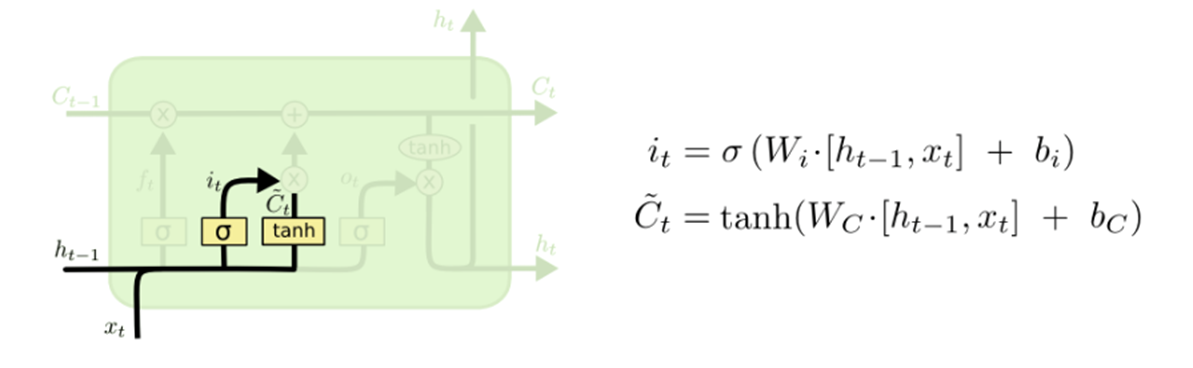我们可以看到其实这些变量在计算上的方法都是类似的，这里值的一提的是我们为什么要选用tanh为激活函数。因为我们的模型在学习的时候，每一次新的输入不一定给机器带来正面的影响，也有可能是负面的影响。所以我们需要的激活函数的返回值有正负。
最后是计算在t时刻的输出ht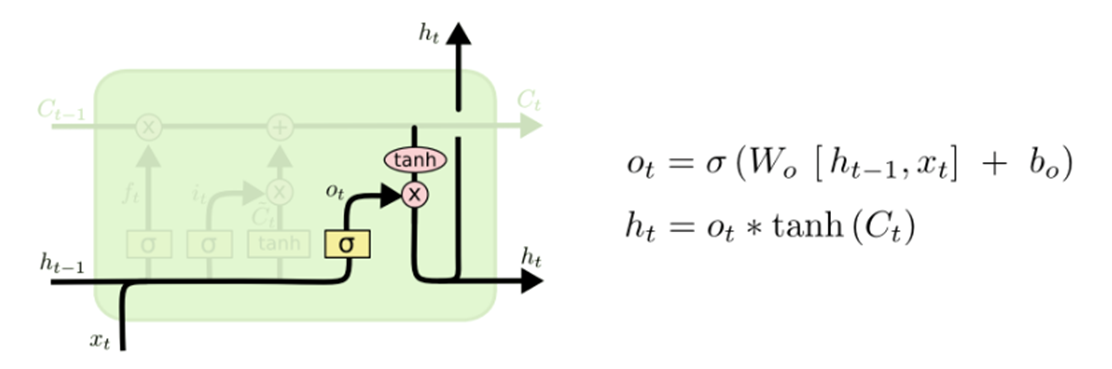我们会发现一个很奇怪的现象，为什么在输出的时候还要乘上一个权重参数Ot
Ot代表你打算用多少Ct激活后的数值做输出的百分比。
举一个生活中的例子，比如你明天要去参加考试了，已经准备的十分充分了，但是你十分低调，有100分的实力但就是不想考100分。虽然不是每个人都这么想，但是我们得给他们这个权力，这个“权力”就是这里的Ot
得出Ctht之后便会传给下一时刻，那么LSTM这一模型就在时间轴上“跑”起来了。

### 如何评价一个分类模型的好坏？

我们通常会建立一个Confusion Matrix：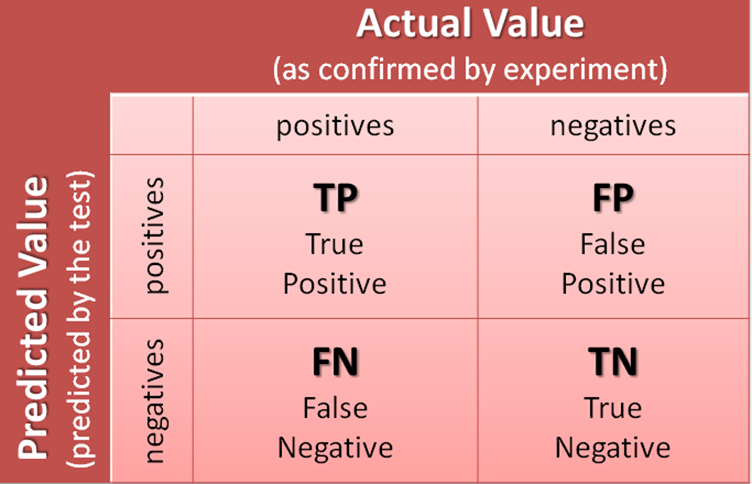这里预测正确的概率是：（TP + TN) / (TP + FP + FN + TN)
延申说一下：
FP：Type I error（本来是错的，被预测成对的）
FN：Type II error （本来是对的，被预测成错的）。

## Coding（实战——气候科学中的EHI）

首先，什么是EHI？
EHI（热浪系数）>0说明热浪对身体有影响，反之则无影响。公式如下图所示：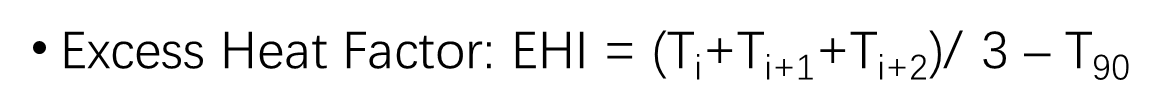先读入数据：

import numpy as np
import matplotlib.pyplot as plt
from netCDF4 import Dataset

file1 = 'pr_Amon_CESM1-CAM5_rcp85_r1i1p1_200601-210012.nc'
file2 = 'tas_Amon_CESM1-CAM5_rcp85_r1i1p1_200601-210012.nc'

ds1 = Dataset(file1,'r')
ds2 = Dataset(file2,'r')

pr = ds1.variables['pr'][:]
tas = ds2.variables['tas'][:]
lon = ds1.variables['lon'][:]
lat = ds1.variables['lat'][:]


我们这边以New York为例：

lon_NY_index =  int((360 - 74)/(360/288))
lat_NY_index = int(40.7 / (180/192)) + 96

tas_NY = tas[:,lat_NY_index,lon_NY_index]
pr_NY = pr[:,lat_NY_index,lon_NY_index]


下面准备数据集：
Tips：LSTM是依赖于时间序列的，所以在进行数据集拆分的时候要注意不能打乱时间！

# prepare data
X = np.hstack((pr_NY[90:-2].reshape(-1,1), tas_NY[90:-2].reshape(-1,1)))
y = np.full([len(tas_NY)-92],np.nan)
for i in range(len(y)):
y[i] = ( np.mean(tas_NY[90+i:90+i+3]) - np.mean(tas_NY[i:i+90])) > 0

X_train = []
y_train = []
for i in range(10,int(len(X)*0.8)):
X_train.append(X[i-10:i,:])
y_train.append(y[i])
X_train, y_train = np.array(X_train), np.array(y_train)

X_test = []
y_test = []
for i in range(int(len(X)*0.8), len(X)):
X_test.append(X[i-10:i,:])
y_test.append(y[i])
X_test, y_test = np.array(X_test), np.array(y_test)


LSTM模型的建立（此处为方便起见我们只建立一层神经网络）：

# build model
from keras.models import Sequential
from keras.layers import LSTM
from keras.layers import Dense

mymodel = Sequential()
mymodel.add(Dense(units=1, activation = 'sigmoid'))


神经网络建立起来之后我们进行训练及预测：

# compile
mymodel.fit(X_train, y_train, epochs = 50, batch_size=50)

# prediction
y_pred = mymodel.predict(X_test)
y_pred = y_pred > 0.5


最后评价此模型：

from sklearn.metrics import confusion_matrix
c = confusion_matrix(y_pred, y_test)
accuracy = (c[0,0]+c[1,1])/(np.sum(c))*100


OK到这里我们基本了解LSTM模型，并且进行了实战。希望对你有帮助 ：）

展开全文Python3 LSTM 人工神经网络
• ## LSTM神经网络图解

万次阅读 2019-07-01 15:51:44
LSTM神经网络图详解 （1）遗忘门，用于计算信息的遗忘（保留）程度，通过sigmoid处理后为0到1的值，1表示全部保留，0表示全部忘记。ft=σ(Wf⋅[ht−1,xt]+bf)f_{t}=\sigma \left ( W_{f}\cdot \left [ h_{t-1},x_{t}...

LSTM神经网络图详解

（1）遗忘门，用于计算信息的遗忘（保留）程度，通过sigmoid处理后为0到1的值，1表示全部保留，0表示全部忘记。 f t = σ ( W f ⋅ [ h t − 1 , x t ] + b f ) f_{t}=\sigma \left ( W_{f}\cdot \left [ h_{t-1},x_{t} \right ] +b_{f}\right )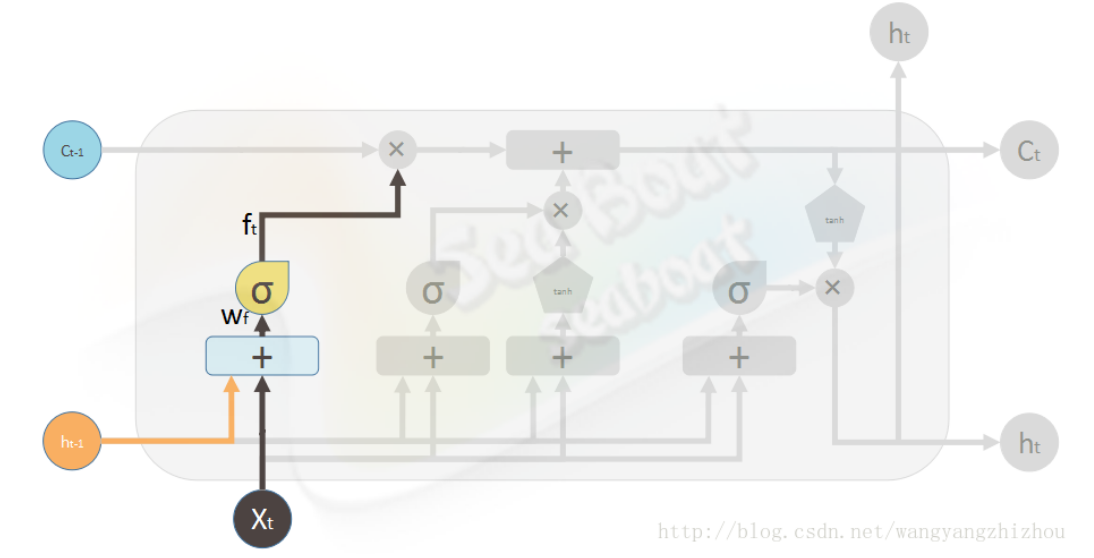(2)输入门，输入门用来计算哪些信息保存到状态单元中,包括两部分信息，一部分是
i t = σ ( W i ⋅ [ h t − 1 , x t ] + b i ) i_{t}=\sigma \left ( W_{i}\cdot \left [ h_{t-1},x_{t} \right ] +b_{i}\right )
该部分可以看成当前输入有多少信息需要保存到单元状态。另一部分是
c t ~ = tanh ⁡ ( W c ⋅ [ h t − 1 , x t ] + b c ) \tilde{c_{t}}=\tanh \left ( W_{c}\cdot \left [ h_{t-1},x_{t} \right ] +b_{c}\right )
该部分用来把当前输入产生的新信息添加到单元状态中。这两部分产生新的记忆状态。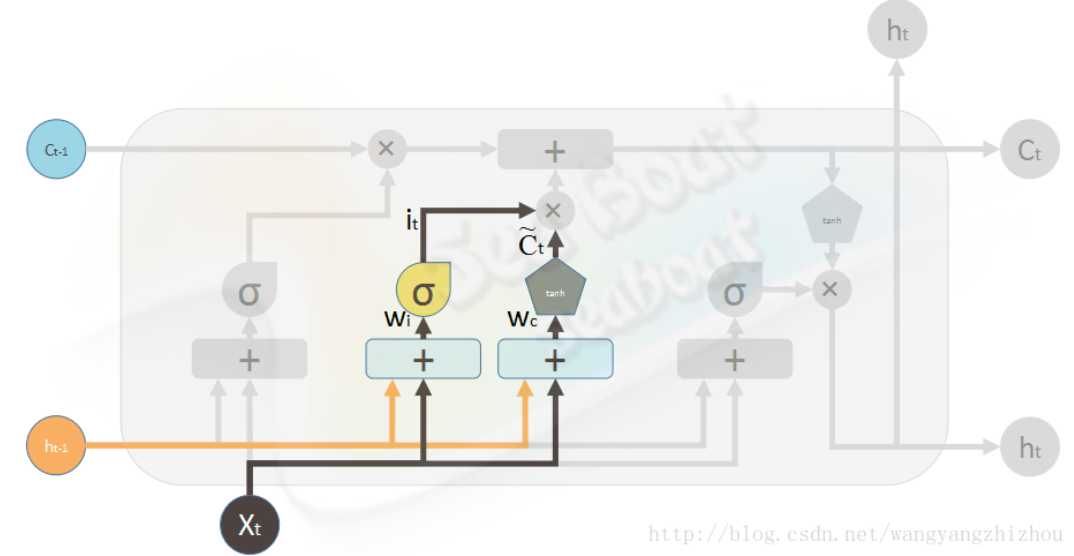由此，当前时刻的单元状态由遗忘门输入和上一时刻状态的积加上输入门两部分的积，即
c t = f t ⋅ c t − 1 + i t ⋅ c ~ t {c_{t}}= f_{t}\cdot c_{t-1}+ i_{t}\cdot \tilde c_{t}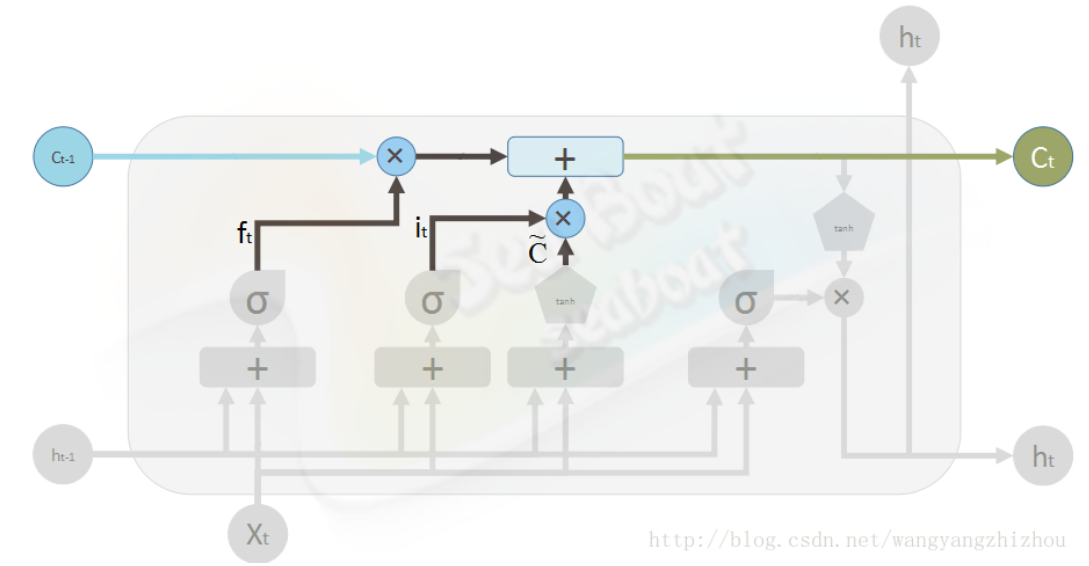（3）输出门，用于计算当前时刻信息被输出的程度。
o t = σ ( W o ⋅ [ h t − 1 , x t ] + b o ) o_{t}=\sigma \left ( W_{o}\cdot \left [ h_{t-1},x_{t} \right ] +b_{o}\right )
h t = o t ⋅ t a n h ( c t ) h_{t}= o_{t}\cdot tanh(c_{t})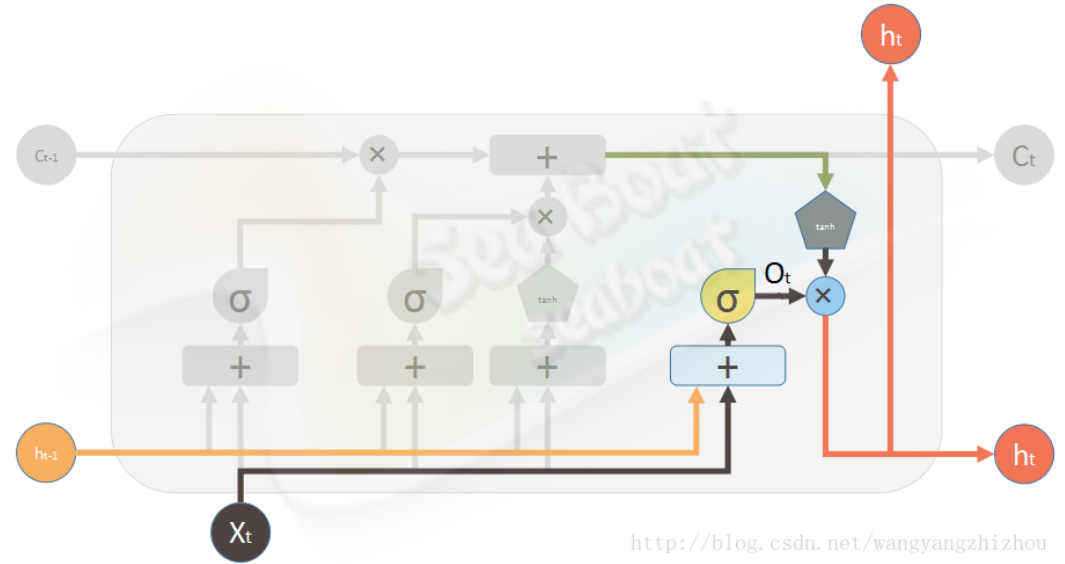整个LSTM神经网络就是如此，下面是李宏毅老师ppt上的，可以进一步了解整个流程。PPT链接循环神经网络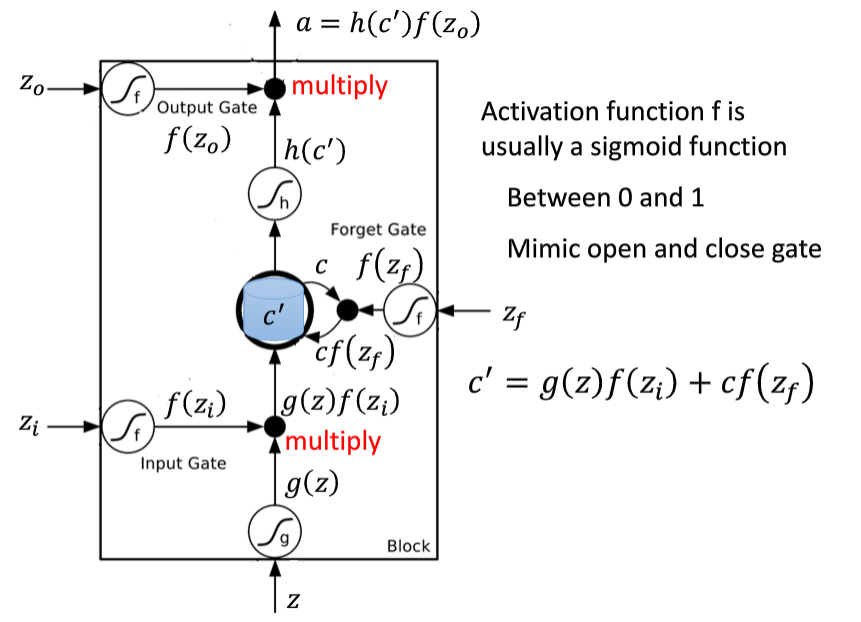对于门（Gate）的理解，这里有很形象的解释深度学习计算模型中“门函数（Gating Function）”的作用

展开全文• 本研究通过改进后的LSTM神经网络模型使用多序列股票预测方法来进行股票价格的涨跌趋势预测. 实验结果证实, 与日数据相比, 周数据的预测效果表现更优, 其中日数据的平均准确率为52.8%, 而周数据的平均准确率为58%, ...
• 本文约2800字，建议阅读10+分钟 ...视频：LSTM神经网络架构和工作原理及其在Python中的预测应用什么是依赖关系？假设您在观看视频时记得前一个场景，或者在阅读一本书时您知道前一章发生了什么。传统的神...本文约2800字，建议阅读10+分钟
本文与你分享如何使用长短期记忆网络（LSTM）来拟合一个不稳定的时间序列。

## 长短期记忆网络——通常称为“LSTM”——是一种特殊的RNN递归神经网络，能够学习长期依赖关系。

视频：LSTM神经网络架构和工作原理及其在Python中的预测应用

什么是依赖关系？

假设您在观看视频时记得前一个场景，或者在阅读一本书时您知道前一章发生了什么。

传统的神经网络无法做到这一点，这是一个主要缺点。例如，假设您想对电影中每一点发生的事件进行分类。目前尚不清楚传统的神经网络如何利用电影中先前事件来推理后来的事件。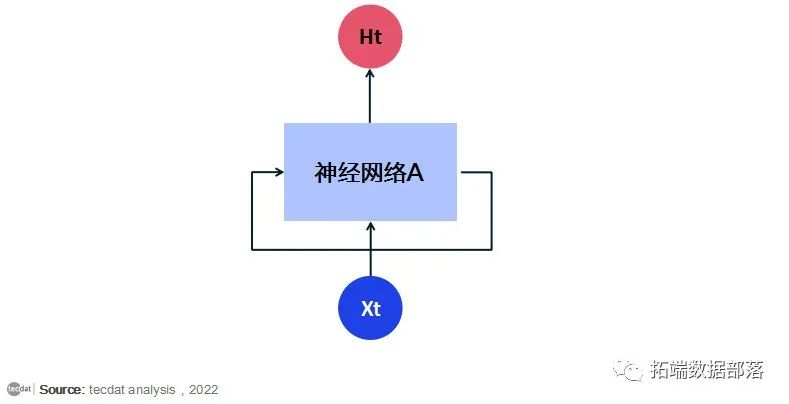递归神经网络解决了这个问题。它们是带有循环的网络，允许信息持续存在。循环神经网络有循环。

在上图中，一大块神经网络，查看一些输入x并输出一个值h. 循环允许信息从网络的一个步骤传递到下一个步骤。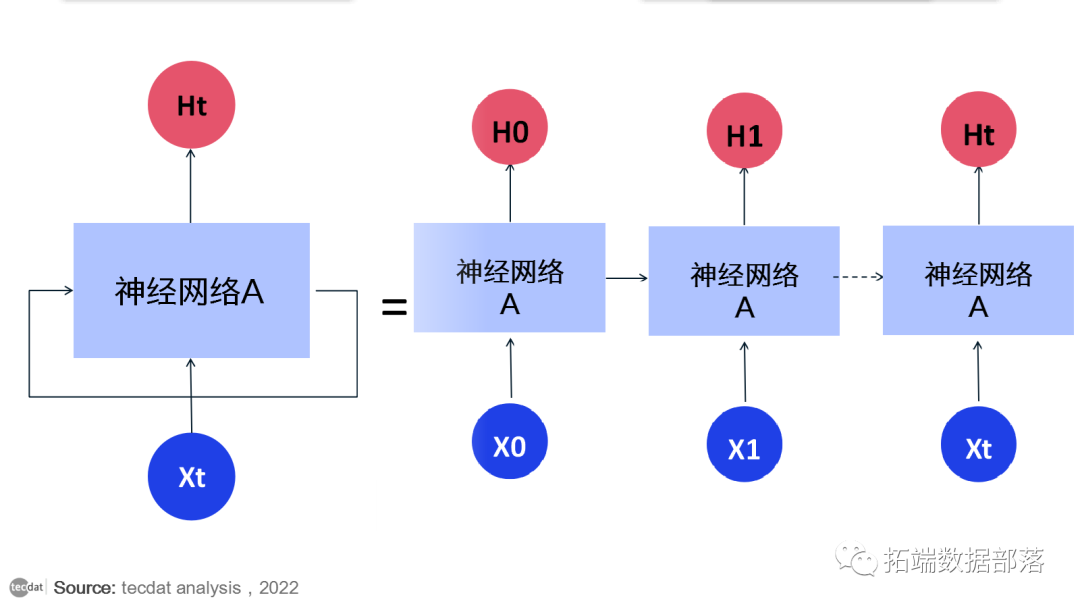这些循环使循环神经网络看起来有点神秘。然而，如果你想得更多，就会发现它们与普通的神经网络并没有什么不同。循环神经网络可以被认为是同一网络的多个副本，每个副本都将消息传递给后继者。考虑一下如果我们展开循环会发生什么：

这种链状性质表明循环神经网络与序列和列表密切相关。它们是用于此类数据的神经网络的自然架构。在过去的几年里，将 RNN 应用于各种问题取得了令人难以置信的成功：语音识别、语言建模、翻译、图像字幕……不胜枚举。这些成功的关键是使用“LSTM”，这是一种非常特殊的循环神经网络，几乎所有基于循环神经网络的令人兴奋的结果都是用它们实现的。本文将探讨的正是这些 LSTM。

长期依赖问题

下面是一个关于如何使用循环神经网络（RNN）来拟合语言模型的例子。

## RNN 的吸引力之一是它们可能能够将先前的信息与当前任务联系起来，例如使用先前的视频帧可能会告知对当前帧的理解。如果 RNN 可以做到这一点，它们将非常有用。但他们可以吗？

有时，我们只需要查看最近的信息即可执行当前任务。例如，考虑一个语言模型试图根据之前的单词预测下一个单词。如果我们试图预测“云在天空”中的最后一个词，我们不需要任何进一步的上下文——很明显下一个词将是天空。在这种情况下，相关信息与所需位置之间的差距很小，RNN 可以学习使用过去的信息。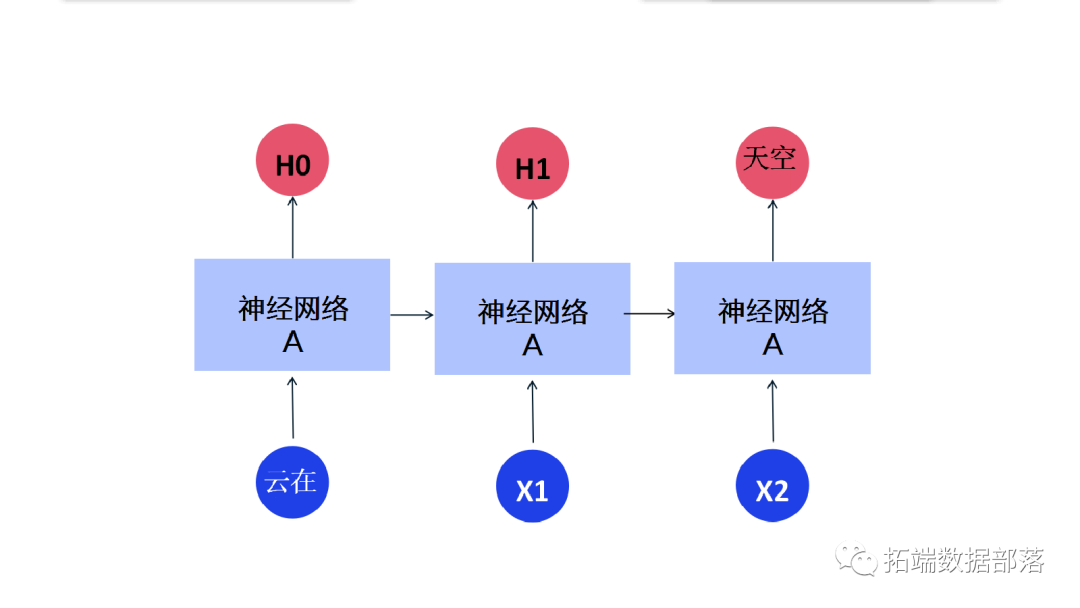但也有我们需要更多上下文的情况。考虑尝试预测文本“我在中国长大……我说地道的中文”中的最后一个词。最近的信息表明，下一个词可能是一种语言的名称，但如果我们想缩小哪种语言的范围，我们需要中国的上下文，从更远的地方。相关信息和需要的点之间的差距完全有可能变得非常大。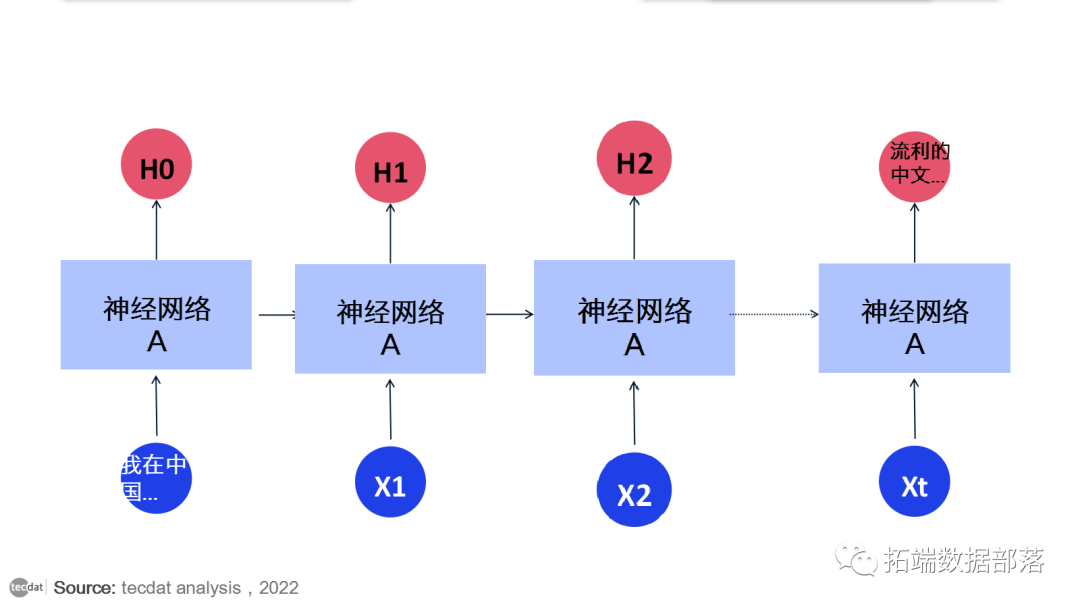随着差距的扩大，RNN 变得无法学习连接信息。

## LSTM 网络

长短期记忆网络——通常称为“LSTM”——是一种特殊的 RNN，能够学习长期依赖关系。它们在解决各种各样的问题时表现出色，现在被广泛使用。LSTM 被明确设计为避免长期依赖问题。长时间记住信息实际上是他们的默认行为，而不是他们难以学习的东西！

所有循环神经网络都具有神经网络的重复模块链的形式。在标准 RNN 中，此重复模块将具有非常简单的结构，例如单个 tanh 层。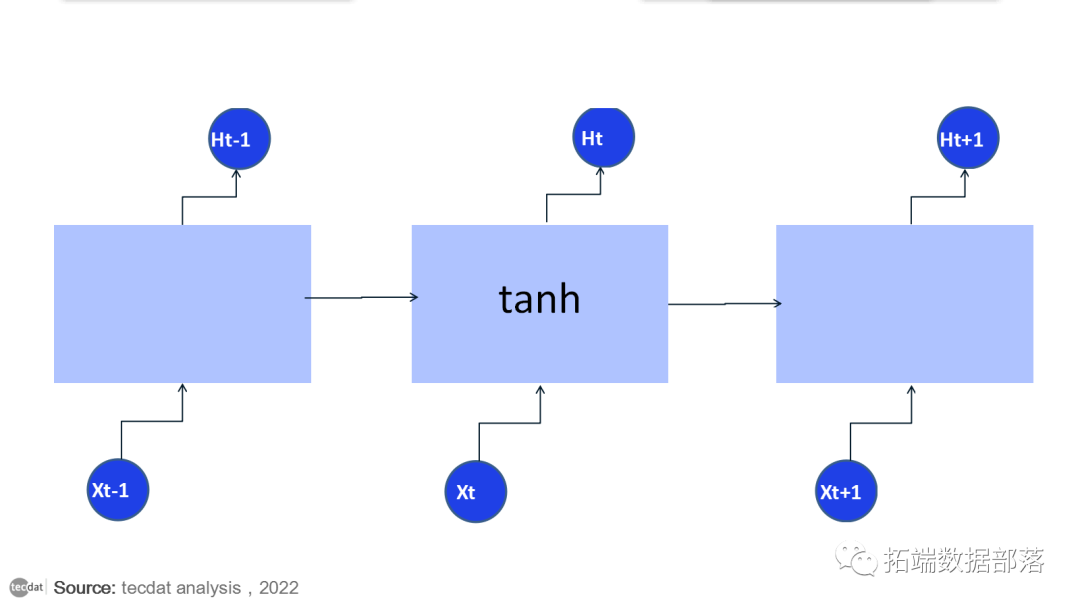LSTM 也有这种链状结构，但重复模块有不同的结构。不是只有一个神经网络层，而是三个部分组成，以一种非常特殊的方式进行交互。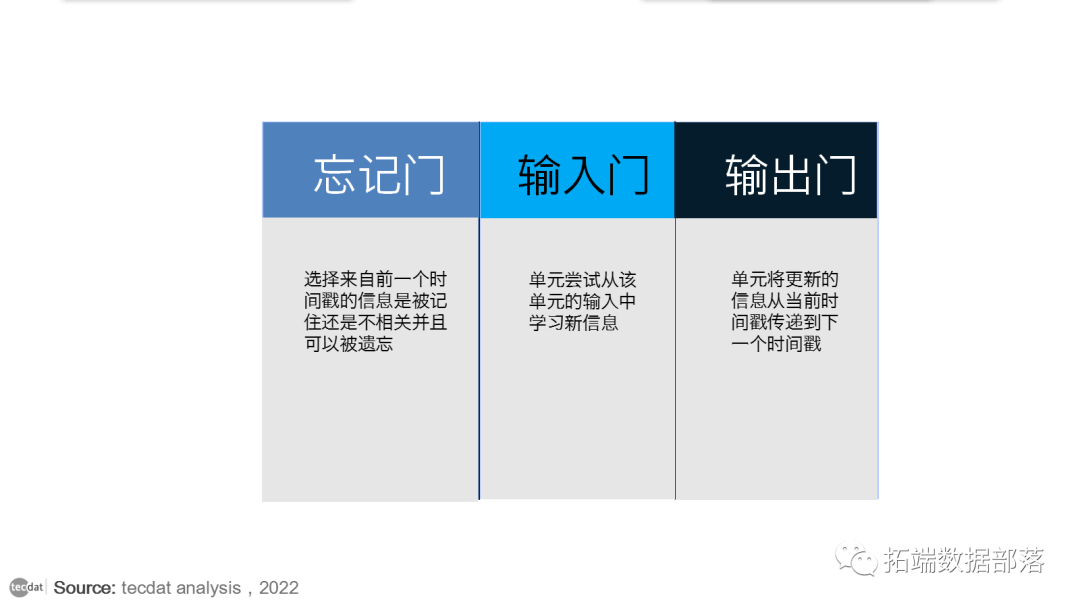LSTM 的工作方式非常类似于 RNN 单元。这是 LSTM 网络的内部功能。LSTM 由三个部分组成，如图所示，每个部分执行一个单独的功能。第一部分选择来自前一个时间戳的信息是被记住还是不相关并且可以被遗忘。在第二部分中，单元尝试从该单元的输入中学习新信息。最后，在第三部分，单元将更新的信息从当前时间戳传递到下一个时间戳。LSTM 单元的这三个部分称为门。第一部分称为忘记门或遗忘门，第二部分称为输入门，最后一部分称为输出门。

Python用LSTM长短期记忆神经网络对不稳定降雨量时间序列进行预测分析

每年的降雨量数据可能是相当不平稳的。与温度不同，温度通常在四季中表现出明显的趋势，而雨量作为一个时间序列可能是相当不平稳的。夏季的降雨量与冬季的降雨量一样多是很常见的。

下面是某地区2020年11月降雨量数据（查看文末了解数据获取方式）的图解。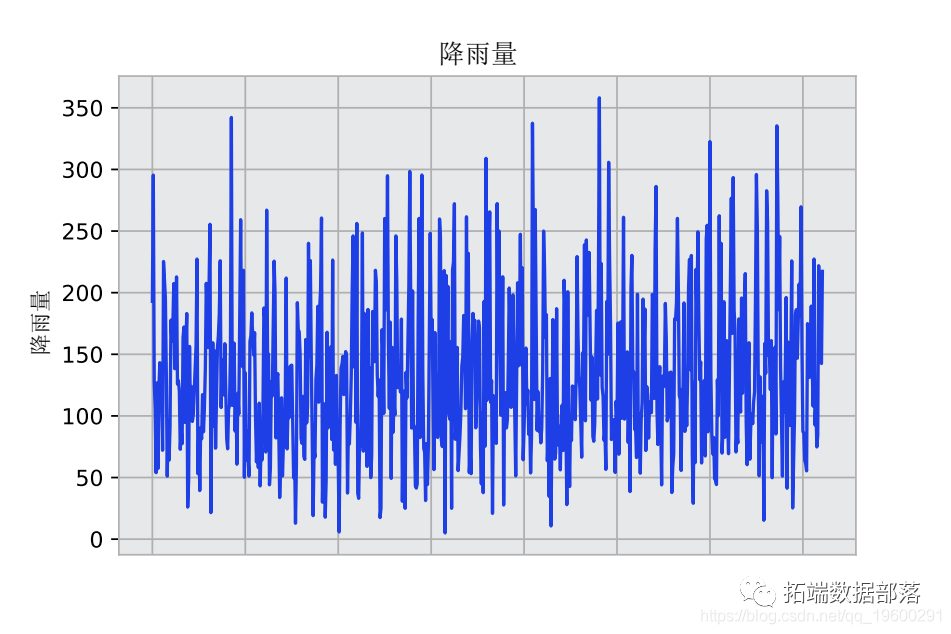作为一个连续的神经网络，LSTM模型可以证明在解释时间序列的波动性方面有优势。

使用Ljung-Box检验，小于0.05的p值表明这个时间序列中的残差表现出随机模式，表明有明显的波动性。

>>> sm.stats.acorr_ljungbox(res.resid, lags=$10$)

### Ljung-Box检验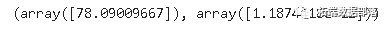### Dickey-Fuller 检验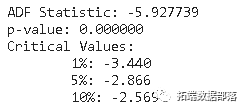# 数据操作和模型配置

该数据集由722个月的降雨量数据组成。

选择712个数据点用于训练和验证，即用于建立LSTM模型。然后，过去10个月的数据被用来作为测试数据，与LSTM模型的预测结果进行比较。

下面是数据集的一个片段。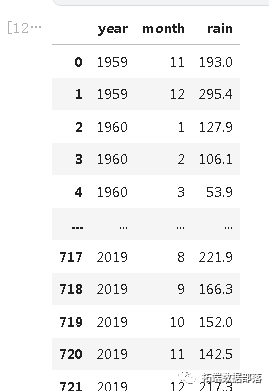然后形成一个数据集矩阵，将时间序列与过去的数值进行回归。

# 形成数据集矩阵

for i in range(len(df)-previous-1):
a = df$i:(i+previous), 0$
dataX.append(a)
dataY.append(df$i + previous, 0$)

然后用MinMaxScaler对数据进行标准化处理。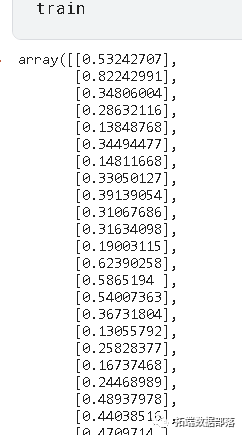将前一个参数设置为120，训练和验证数据集就建立起来了。作为参考，previous = 120说明模型使用从t - 120到t - 1的过去值来预测时间t的雨量值。

前一个参数的选择要经过试验，但选择120个时间段是为了确保识别到时间序列的波动性或极端值。

# 训练和验证数据的划分
train_size = int(len(df) * 0.8)
val\_size = len(df) - train\_size
train, val = df$0:train\_size,:$, df$train\_size:len(df),:$# 前期的数量
previous = 120

然后，输入被转换为样本、时间步骤、特征的格式。

# 转换输入为$样本、时间步骤、特征$。
np.reshape(X_train, (shape$0$, 1, shape$1$))

# 模型训练和预测

该模型在100个历时中进行训练，并指定了712个批次的大小（等于训练和验证集中的数据点数量）。

# 生成LSTM网络
model = tf.keras.Sequential()
# 列出历史中的所有数据
print(history.history.keys())
# 总结准确度变化
plt.plot(history.history$'loss'$)

下面是训练集与验证集的模型损失的关系图。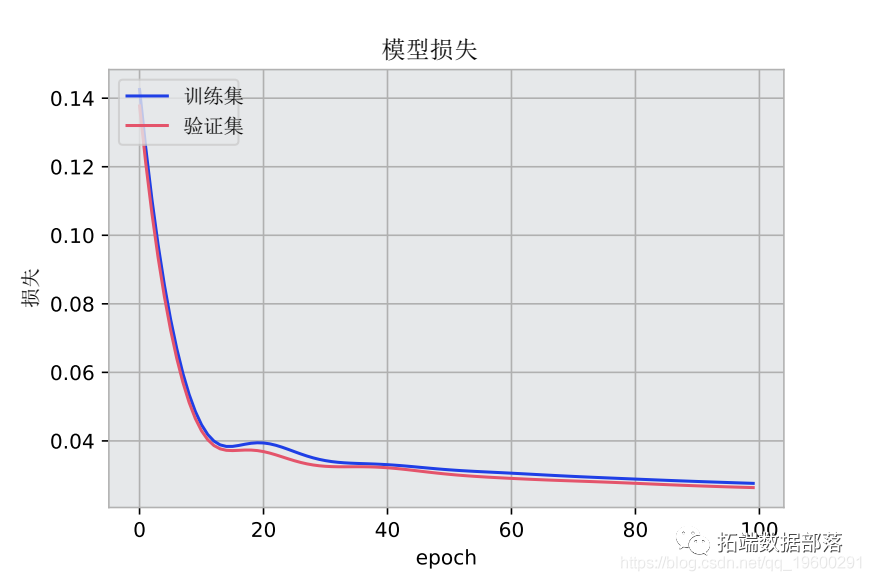预测与实际降雨量的关系图也被生成。

# 绘制所有预测图
plt.plot(valpredPlot)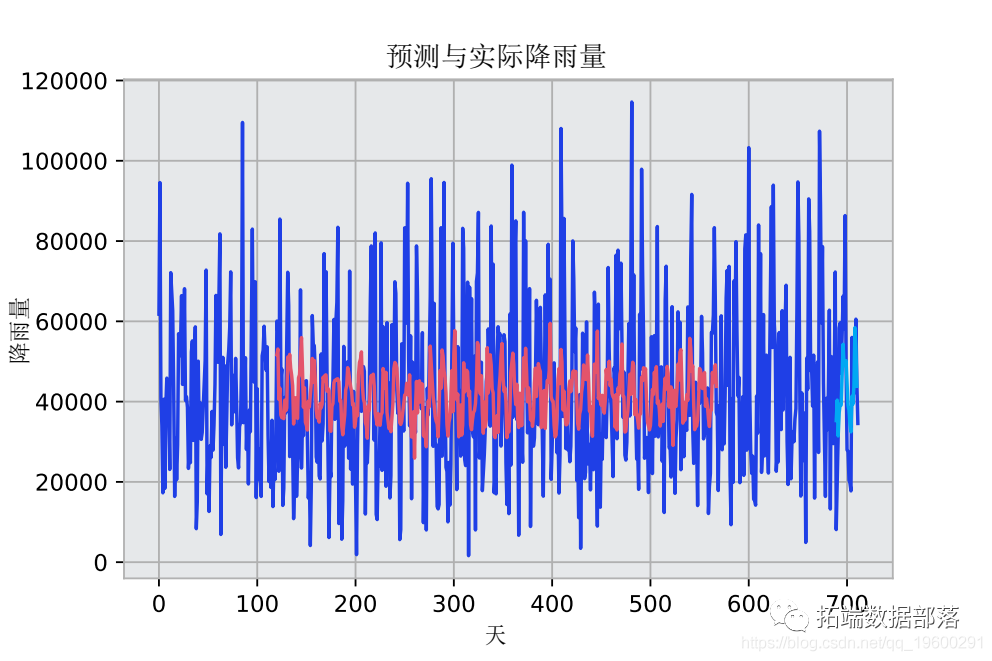预测结果在平均方向准确性（MDA）、平均平方根误差（RMSE）和平均预测误差（MFE）的基础上与验证集进行比较。

mda(Y_val, predictions)0.9090909090909091
>>> mse = mean\_squared\_error(Y_val, predictions)
>>> rmse = sqrt(mse)
>>> forecast_error
>>> mean\_forecast\_error = np.mean(forecast_error)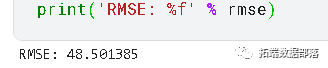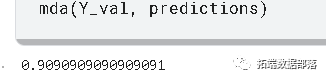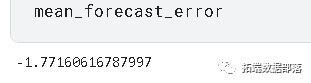• MDA: 0.909

• RMSE: 48.5

• MFE: -1.77

# 针对测试数据进行预测

虽然验证集的结果相当可观，但只有将模型预测与测试（或未见过的）数据相比较，我们才能对LSTM模型的预测能力有合理的信心。

如前所述，过去10个月的降雨数据被用作测试集。然后，LSTM模型被用来预测未来10个月的情况，然后将预测结果与实际值进行比较。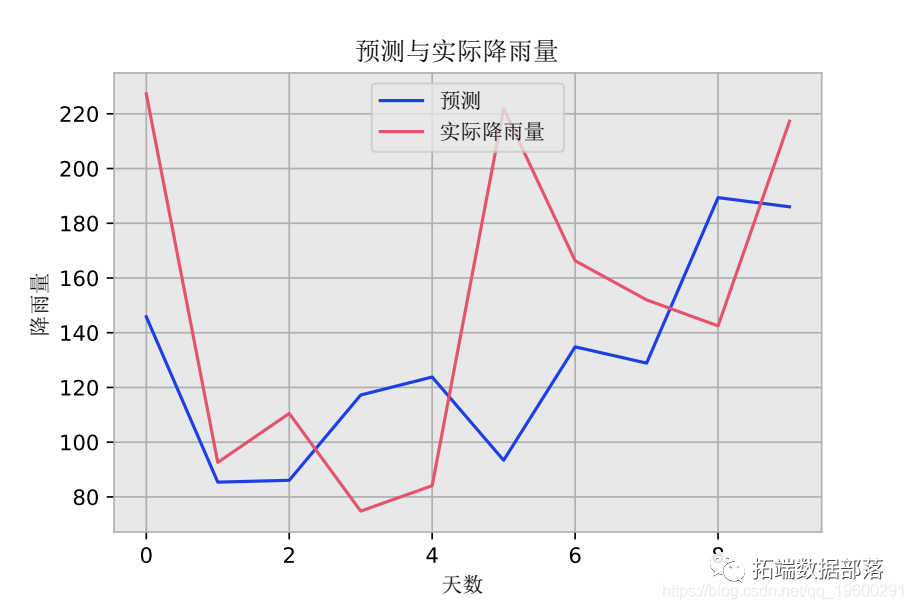至t-120的先前值被用来预测时间t的值。

# 测试（未见过的）预测
np.array(\[tseries.iloctseries.iloc,t

获得的结果如下：

• MDA: 0.8

• RMSE: 49.57

• MFE: -6.94

过去10个月的平均降雨量为148.93毫米，预测精度显示出与验证集相似的性能，而且相对于整个测试集计算的平均降雨量而言，误差很低。

# 结论

在这个例子中，你已经看到：

• 如何准备用于LSTM模型的数据

• 构建一个LSTM模型

• 如何测试LSTM的预测准确性

• 使用LSTM对不稳定的时间序列进行建模的优势本文摘选《Python用LSTM长短期记忆神经网络对不稳定降雨量时间序列进行预测分析》，点击“阅读原文”获取全文完整资料。

编辑：黄继彦展开全文神经网络 python 机器学习 人工智能
• ## LSTM神经网络详解

万次阅读 多人点赞 2020-10-12 20:43:11
长短时记忆网络(Long Short Term Memory Network, LSTM)，是一种改进之后的循环神经网络，可以解决RNN无法处理长距离的依赖的问题，目前比较流行。 长短时记忆网络的思路： 原始 RNN 的隐藏层只有一个状态，即h，它...
• 参考... ''' 用tensorflow实现递归循环网络LSTM） ''' from __future__ import print_function import tensorflow as tf from tensorflow.contrib import rnn #导入MINIST数据...tensorflow LSTM
• Python基于LSTM神经网络的时间序列预测 使用LSTM神经网络进行时间序列数据预测分析。 基于Tensorflow框架、Kerase接口开发网络模型。 包含数据清洗，数据特征提取，数据建模，数据预测。 Python基于LSTM神经...
• 相反，我们希望尝试使用Tensorflow构建组合的CNN-LSTM神经网络模型，以对Twitter数据进行情感分析。 依存关系 sudo -H pip install -r requirements.txt 运行代码 在train.py上，更改变量MODEL_TO_RUN = {0或1} 0 ...
• ## 简单理解LSTM神经网络

万次阅读 多人点赞 2019-05-23 15:39:27
在传统神经网络中，模型不会关注上一时刻的处理会有什么信息可以用于下一时刻，每一次都只会关注当前时刻的处理。举个例子来说，我们想对一部影片中每一刻出现的事件进行分类，如果我们知道电影前面的事件信息，那么...
• 1997年，Hochreiter和Schmidhuber一起提出长短期记忆神经网络,即LSTM神经网络LSTM神经网络很好地解决了RNN中存在的梯度消失和梯度爆炸问题。与RNN相比，它可以记忆更长时间步上的信息。2013年，Graves等对LSTM神经...matlab
• 基于LSTM神经网络的黑色金属期货套利策略模型.pdf...